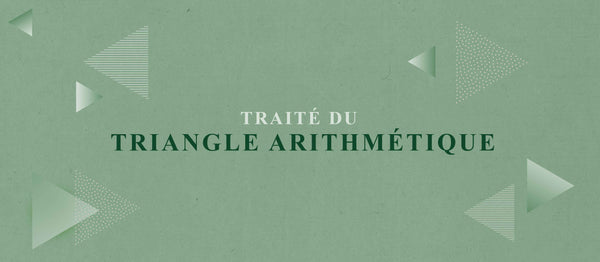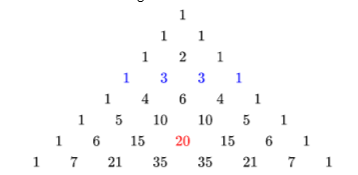Traité du triangle arithmétique

Blaise Pascal was a seventeeth century French mathematician (also physicist, inventor, writer, and Catholic theologian...) who made important contributions towards concepts such as pressure, vacuums and pioneered calculating machines – all while still a teenager. His name may sound familiar, and for good reason. In 1654, Pascal cemented his place in the history of mathematics with the publication of his ‘Traité du triangle arithmétique’, a beautiful creation that we now call ‘Pascal’s triangle’.The triangle is a series of numbers presented in a triangular shape where each number is calculated as the summation of the two numbers above, all starting from 1 (and invisible 0s). The symmetry of the triangle that follows is appealing; however, there are several other interesting and often beautiful patterns to be found! Here are three of the most significant (there are hundreds more…):

The Binomial Coefficient

Okay, so this isn’t such a coincidence: the original construction of the triangle was motivated by Pascal’s work on his coefficient.The connection with the triangle is clear when taking an example, say the expansion of (x+1) to the power of 4. Then the number before each power of x (in descending order of powers) follows the exact same numbers on the 4th row of the triangle.

The applications of the coefficient are not limited to calculus as the function also features heavily in probability where it is more commonly referred to as ‘n choose k’. This is because it also represents the number of unique subsets of size k from a set of size n. For example, if you have 8 friends and must choose just 5 to go to the pub with, simply sketch out the triangle quickly and see that there are 56 possible choices for which 5 friends (the value in the 9th row and 6th column of the triangle). Unfortunately Pascal gave no formula for how to choose…

The Fibonacci Sequence

A similar popular mathematical concept, this time a sequence where each term is defined as the summation of the previous two, the Fibonacci Sequence has several interesting patterns itself. These two classical maths phenomena are beautifully linked as the sequence can also be generated by summing diagonals of the Pascal triangle (as shown).

Diagonals

The sequence of numbers along each diagonal of the triangle presents its own pattern. Firstly, the outer diagonal of all 1s, and then adjacent to that the sequence of natural numbers. The third diagonal presents the numbers 1,3,6,10 ,15.. which most will recognise as the sequence of triangle numbers. The fourth diagonal contains the lesser known tetrahedral numbers and fifth the pentatope numbers; clearly, there is also a pattern in the patterns!